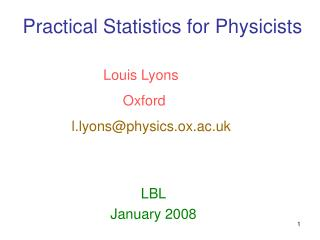DownloadDownload PresentationPractical Statistics for Physicists

# Practical Statistics for Physicists

Télécharger la présentation## Practical Statistics for Physicists

- - - - - - - - - - - - - - - - - - - - - - - - - - - E N D - - - - - - - - - - - - - - - - - - - - - - - - - - -
##### Presentation Transcript

1. Practical Statistics for Physicists Louis Lyons Oxford l.lyons@physics.ox.ac.uk LBL January 2008

2. PARADOX Histogram with 100 bins Fit 1 parameter Smin: χ2 with NDF = 99 (Expected χ2 = 99 ± 14) For our data, Smin(p0) = 90 Is p1 acceptable if S(p1) = 115? • YES. Very acceptable χ2 probability • NO. σp from S(p0 +σp) = Smin +1 = 91 But S(p1) – S(p0) = 25 So p1 is 5σ away from best value

3. Comparing data with different hypotheses

4. Choosing between 2 hypotheses Possible methods: Δχ2 lnL–ratio Bayesian evidence Minimise “cost”

5. Learning to love the Error Matrix • Resume of 1-D Gaussian • Extend to 2-D Gaussian • Understanding covariance • Using the error matrix Combining correlated measurements • Estimating the error matrix

6. Element Eij - <(xi – xi) (xj – xj)> Diagonal Eij = variances Off-diagonal Eij = covariances

7. N.B. Small errors

8. Mnemonic: (2*2) = (2*4) (4*4) (4*2) r c r c 2 = x_a, x_b 4 = p_i, p_j………

9. Difference between averaging and adding Isolated island with conservative inhabitants How many married people ? Number of married men = 100 ± 5 K Number of married women = 80 ± 30 K Total = 180 ± 30 K Weighted average = 99 ± 5 K CONTRAST Total = 198 ± 10 K GENERAL POINT: Adding (uncontroversial) theoretical input can improve precision of answer Compare “kinematic fitting”

10. Small error xbest outside x1 x2 ybest outside y1  y2

11. b y a x

12. Conclusion Error matrix formalism makes life easy when correlations are relevant

13. Tomorrow • Upper Limits • How Neural Networks work